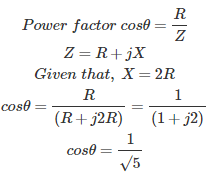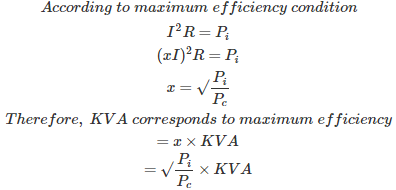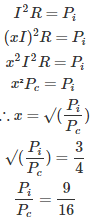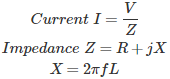# MCQs on Electrical Machines

##### Page 41 of 114. Go to page 1 2 3 4 5 6 7 8 9 10 11 12 13 14 15 16 17 18 19 20 21 22 23 24 25 26 27 28 29 30 31 32 33 34 35 36 37 38 39 40 41 42 43 44 45 46 47 48 49 50 51 52 53 54 55 56 57 58 59 60 61 62 63 64 65 66 67 68 69 70 71 72 73 74 75 76 77 78 79 80 81 82 83 84 85 86 87 88 89 90 91 92 93 94 95 96 97 98 99 100 101 102 103 104 105 106 107 108 109 110 111 112 113 114
01․ Open circuit test on transformer gives
eddy current loss
hysteresis loss
copper loss
both 1and 2

The no load current drawn by the primary is around 2 % to 6 % of rated current. Therefore copper loss of primary is very low and can be neglected. As the copper loss is negligible, the watt-meter reading is considered as iron loss or constant loss i.e eddy current loss and hysteresis loss.

02․ Open circuit in a transformer is prefered with
rated transformer voltage
rated transformer current
direct current
high frequency supply

As the name implies, high voltage side is left open and the rated voltage is applied to the low voltage side to get the constant loss, because constant loss depends on the supply voltage. Therefore rated voltage is applied at the low voltage side for no-load test.

03․ In a transformer
both OC and SC tests are conducted on LV side
OC test is conducted on LV side and SC test is conducted on HV side
OC test is conducted on HV side and SC test is conducted on LV side
both Oc and SC tests are conducted on HV side

In open circuit test HV is left open on no load and the rated voltage is applied to the low voltage side to get the constant losses. Rated voltage on LV side is lesser than the HV side. For convenience and better readings, OC test is always preferred to be conducted on LV side and leaving HV side open. In SC test, as name implies LT winding is short circuited and rated current should be ensured in the primary winding. As rated currents are low on HV side, SC test is preferred on HV side and short circuiting LV side.

04․ In a single phase transformer the magnitude of leakage reactance is twice that of resistance of both primary and secondary. With secondary short circuited, the input power factor is
1/√2
1/√5
2/√5
1/305․ For a transformer to be tested at full load conditions but consuming only losses from the main, we do
OC and SC tests
back to back test
none of the above

In OC test there will be only iron losses in the transformer, in SC test there will be only copper loss but under practical conditions both losses occur simultaneously and the temperature rise is due to both losses. To determine iron loss, copper loss and temperature rise for a designated period back to back test or sumpner's test is conducted.

06․ If Pi and Pc represent core and full load copper loss respectively, the maximum KVA delivered to load corresponding to maximum efficiency is equal to rated KVA is
Pi/Pc
(Pi/Pc)2
√(Pi/Pc)
Pc/Pi

Condition for maximum efficiency is, Copper loss Pc = Iron loss Pi I2 R = Pi A transformer can be operated at any load but maximum efficiency occurs at a particular load condition only. Let x be that load factor corresponds to maximum efficiency.07․ A transformer has maximum efficiency at 3/4 load. The ratio of iron loss and full load copper loss is
4/3.
3/4.
9/16.
16/9.

Condition for maximum efficiency is, Copper loss Pc = Iron loss Pi I² R = Pi A transformer can be operated at any load but maximum efficiency occurs at a particular load condition only. Let x be that load factor corresponds to maximum efficiency. According to maximum efficiency condition x = Condition for maximum efficiency, Given that, maximum efficiency will occurs at 3/4 load.08․ When a short circuit test on a transformer is performed at 25 V, 50 Hz, the drawn current is I1. If the test is performed by 25 V and 25 Hz and power drawn current is I2, then
I1 > I2
I1 < I2
I1 = I2
none of the aboveAs frequency decreases, reactance will decrease and the current drawn I2 will be more than I1.

09․ A 2 KVA transformer has iron loss of 150 W and full load copper loss of 250 W. The maximum efficiency of the transformer would occur when the total loss is
500 W
400W
250 W
300 W

The condition for maximum efficiency is, Full load copper loss Pc = Iron loss, Pi and copper to be reduced to the value of iron loss, Total loss P = Pc + Pi = 150 + 150 = 300 W

10․ Silicon content in steel lamination is kept within 5% as it
increases hysteresis loss.
Increases cost.
makes the material brittle.
all of the above.

Steel is considered to be having good permeability and mechanical properties. But it has high conductivity. To reduce conductivity by not destroying magnetic properties, alloying with silicon is done by 3.5 % to 4 %. More silicon percentage will destroy the mechanical properties; this will make the material brittle.

<<<3940414243>>>